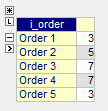# Model category mapping of items

Very often, you will find yourselves having to define a certain property for an item based on its category. For example, cost(\$) of an item based on the size of that item (small, medium, or large). This is similar to using a `lookup` function in Excel to create a map from the size-cost table to the list of items. This article will show you how to model such a category mapping in AIMMS.

## Use Case

A simple example case is if you have a list of different orders for an item (of three different sizes), you know the price per unit for each size and you want to calculate the cost of each order.

Size

Price(\$)

Small

3

Medium

5

Large

7

The accurate mapping from the above table to the below table should be 3, 5, 7, 7, 3. How do you lookup the corresponding price for each Order according to the OrderSize ?

Order

OrderSize

Cost (\$)

Order 1

Small

Order 2

Medium

Order 3

Large

Order 4

Large

Order 5

Small

## Solution

One way to do this is to define OrderSize as an element parameter which lets us assign a set element to an indexed parameter. A parameter type identifier is used to store numerical values, an element parameter type identifier is used to store elements of a set, and a string parameter type identifier is used to store string values like the name suggests. Remember to use an element parameter even if your set contains numerical elements.

In the `Category Modelling Example AIMMS Project`, you have sets `sSizes` and `sOrders` with `s` and `o` as indices respectively. Price per size is stored in the indexed parameter `pPrice(s)`.

Order size for each order is stored in an element parameter `epOrderSize(o)` which has the attribute range defined as as `sSizes`. The range attribute is mandatory for an element parameter and it should be defined as the set which contains the elements to be stored in that element parameter. In this example, since order sizes small, medium, and large are elements in the set `sSizes`, the range for `epOrderSize` is `sSizes`.

Now, the cost of each order `pOrderCost(o)` can be calculated using the following definition.

```pPrice(epOrderSize(o))
```

For each o, the corresponding s is mapped using the element parameter epOrderSize(o). For example, the evaluation of pOrderCost(Order 1) will be as follows

• pPrice(epOrderSize(Order 1))

• pPrice(Small)

• 3

The resulting data page of the parameter `pOrderCost(o)` will look as below, with the expected mapping.